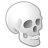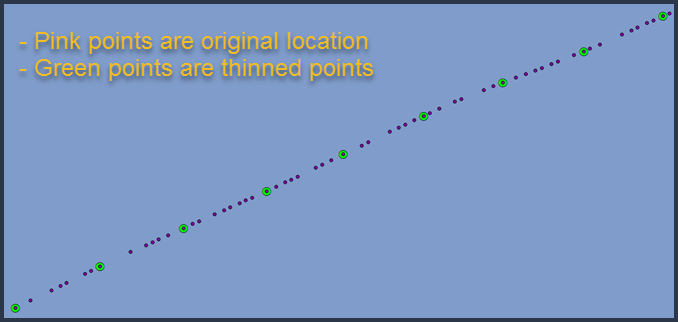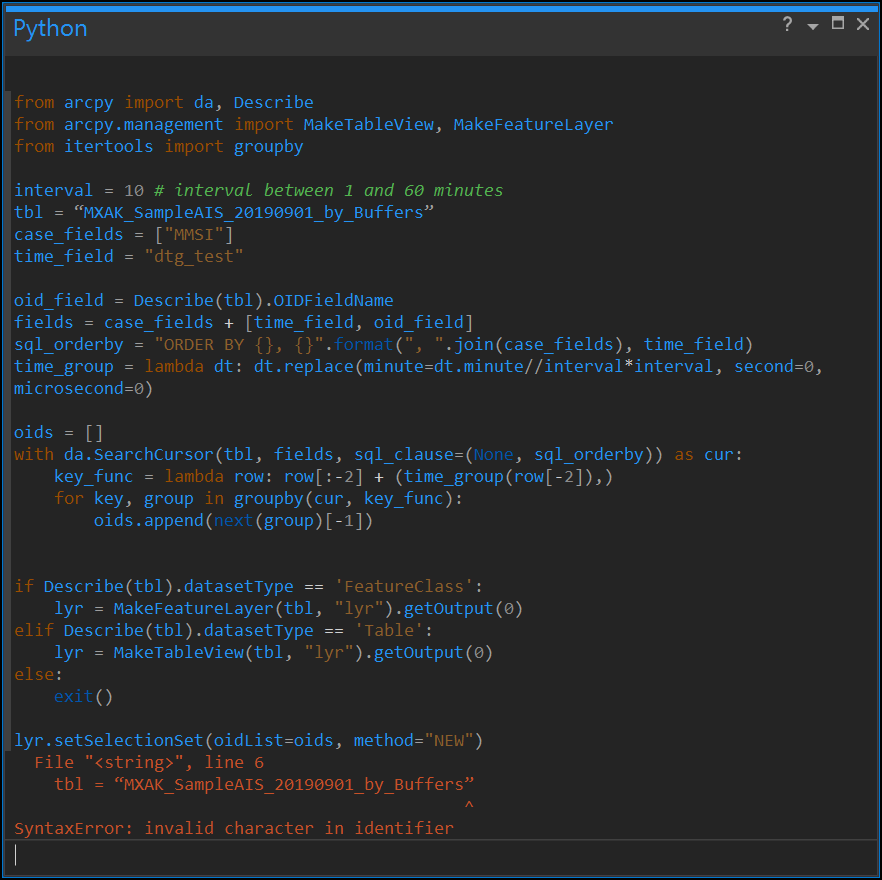# Thinning points based on a time interval

5528
10
04-10-2020 10:38 AMNew Contributor III

Not sure of the etiquette here, but I am reposting this question on the ArcGIS Pro feed, as I didn't get any responses under the ArcMap heading.

************

Hi-- I want to reduce the number of points in vessel tracklines based on a time interval from a datetimestamp value. I can reduce the number of points based on distance using the 'Delete Identical' tool, but I'd like to be able to reduce the number of points by selecting points based on a user defined interval, like a two or five minute interval, and culling the extraneous points. I have not found any obvious way to do this with the built in tools in ArcMap or Pro, but I'm hoping someone else has worked through this and can suggest a workflow or solution. I am still more comfortable working in ArcMap, but can work in Pro if the solution lies there.

Here is simplified version of what the tabular data look like. The highlighted rows show what records would be selected if I chose four minute interval. It makes sense that some rounding of the times may be needed to standardize the time breaks.Here is what the desired output might look like.Thanks!

1 Solution

Accepted SolutionsbyMVP Esteemed Contributor

The following in the interactive Python window will create a new feature layer or table view and make a selection against it:

``````from arcpy import da, Describe
from arcpy.management import MakeTableView, MakeFeatureLayer
from itertools import groupby

interval = 3 # interval between 1 and 60 minutes
tbl = # path to feature class or table
case_fields = ["Vessel"]
time_field = "DTS_DateField"

oid_field = Describe(tbl).OIDFieldName
fields = case_fields + [time_field, oid_field]
sql_orderby = "ORDER BY {}, {}".format(", ".join(case_fields), time_field)
time_group = lambda dt: dt.replace(minute=dt.minute//interval*interval, second=0, microsecond=0)

oids = []
with da.SearchCursor(tbl, fields, sql_clause=(None, sql_orderby)) as cur:
key_func = lambda row: row[:-2] + (time_group(row[-2]),)
for key, group in groupby(cur, key_func):
oids.append(next(group)[-1])

if Describe(tbl).datasetType == 'FeatureClass':
lyr = MakeFeatureLayer(tbl, "lyr").getOutput(0)
elif Describe(tbl).datasetType == 'Table':
lyr = MakeTableView(tbl, "lyr").getOutput(0)
else:
exit()

lyr.setSelectionSet(oidList=oids, method="NEW")
‍‍‍‍‍‍‍‍‍‍‍‍‍‍‍‍‍‍‍‍‍‍‍‍‍‍‍‍‍``````

The above selects the first record in a 3-minute interval.  You can pick whatever minute interval you want.  It is also possibly to get fancier with record selection within the time interval, but selecting the first is the simplest.

10 RepliesbyMVP Esteemed Contributor

You could do a cumulative sum of the field, take the integer of that and the modulos

A poor example follows... 0 represents the points to keep.  Query for those.

``````a = np.random.randint(0, 10, 20)
s = np.cumsum(a)
m = s % 4

a
array([7, 5, 3, 3, 5, 1, 2, 7, 7, 6, 9, 0, 6, 7, 6, 6, 4, 6, 7, 9])

s
array([  7,  12,  15,  18,  23,  24,  26,  33,  40,  46,  55,  55,  61,
68,  74,  80,  84,  90,  97, 106], dtype=int32)

m
array([3, 0, 3, 2, 3, 0, 2, 1, 0, 2, 3, 3, 1, 0, 2, 0, 0, 2, 1, 2],
dtype=int32)

# ---- sample where values are '0'‍‍‍‍‍‍‍‍‍‍‍‍‍‍‍‍``````byMVP Esteemed Contributor

cumulative sum options... python parser.

``````"""-----------------------------------------
Return the cumulative sum of a field
cumulative(field_name)  # enter into the expression box
"""
old = 0   # include this line
def cumulative(new):
'''accumulate values'''
global old
if old >= 0:
old = old + new
else:
old = new
return old

#  or

total = 0
def cumsum(in_field):
global total
total += in_fieldNew Contributor III

Thanks for the input, Dan! My computer is in for much needed maintenance, but I'll ponder and try this approach when I am back at it next week.byMVP Esteemed Contributor

The following in the interactive Python window will create a new feature layer or table view and make a selection against it:

``````from arcpy import da, Describe
from arcpy.management import MakeTableView, MakeFeatureLayer
from itertools import groupby

interval = 3 # interval between 1 and 60 minutes
tbl = # path to feature class or table
case_fields = ["Vessel"]
time_field = "DTS_DateField"

oid_field = Describe(tbl).OIDFieldName
fields = case_fields + [time_field, oid_field]
sql_orderby = "ORDER BY {}, {}".format(", ".join(case_fields), time_field)
time_group = lambda dt: dt.replace(minute=dt.minute//interval*interval, second=0, microsecond=0)

oids = []
with da.SearchCursor(tbl, fields, sql_clause=(None, sql_orderby)) as cur:
key_func = lambda row: row[:-2] + (time_group(row[-2]),)
for key, group in groupby(cur, key_func):
oids.append(next(group)[-1])

if Describe(tbl).datasetType == 'FeatureClass':
lyr = MakeFeatureLayer(tbl, "lyr").getOutput(0)
elif Describe(tbl).datasetType == 'Table':
lyr = MakeTableView(tbl, "lyr").getOutput(0)
else:
exit()

lyr.setSelectionSet(oidList=oids, method="NEW")
‍‍‍‍‍‍‍‍‍‍‍‍‍‍‍‍‍‍‍‍‍‍‍‍‍‍‍‍‍``````

The above selects the first record in a 3-minute interval.  You can pick whatever minute interval you want.  It is also possibly to get fancier with record selection within the time interval, but selecting the first is the simplest.New Contributor III

Thanks Joshua! I just tested it out and it works great for thinning points at whatever minute interval I choose. This could be a game changer for me, assuming I can handle some of the massive datasets I have.New Contributor III

Hey Joshua-- I'm not sure if you'll be willing to chime in on this, but strangely I am not able to run this script today using the same data, project and syntax as last week. I am not well versed in python (I plan to take esri's online course soon!), so I'm guessing the solution is simple and just reflects my ignorance. If you want, I can send you a small sample dataset and you can see what I am working with. But I understand if you don't have the time or desire to offer more me free help!

Below is the python script that I successfully last week, where the input point layer (tbl = “test_points”) lives in the project's default database (C:\GIS\geodata\USA\Vessel Presence Study\AIS Data\MXAK Data\MXAK Sample Data.gdb).

``````from arcpy import da, Describe
from arcpy.management import MakeTableView, MakeFeatureLayer
from itertools import groupby

interval = 10 # interval between 1 and 60 minutes
tbl = “test_points”
case_fields = ["MMSI"]
time_field = "dtg_test"

oid_field = Describe(tbl).OIDFieldName
fields = case_fields + [time_field, oid_field]
sql_orderby = "ORDER BY {}, {}".format(", ".join(case_fields), time_field)
time_group = lambda dt: dt.replace(minute=dt.minute//interval*interval, second=0, microsecond=0)

oids = []
with da.SearchCursor(tbl, fields, sql_clause=(None, sql_orderby)) as cur:
key_func = lambda row: row[:-2] + (time_group(row[-2]),)
for key, group in groupby(cur, key_func):
oids.append(next(group)[-1])

if Describe(tbl).datasetType == 'FeatureClass':
lyr = MakeFeatureLayer(tbl, "lyr").getOutput(0)
elif Describe(tbl).datasetType == 'Table':
lyr = MakeTableView(tbl, "lyr").getOutput(0)
else:
exit()

lyr.setSelectionSet(oidList=oids, method="NEW") ‍‍‍‍‍‍‍‍‍‍‍‍‍‍‍‍‍‍‍‍‍‍‍‍‍‍‍‍‍‍‍‍‍‍‍‍‍‍‍‍‍‍‍‍‍‍‍‍‍‍‍‍‍‍‍‍‍‍``````

I get the following syntax error, even though this ran before:One related question, assuming I can get the script to runs: can I write out the full path for 'tbl', as in

'tbl = C:\GIS\geodata\USA\Vessel Presence Study\AIS Data\MXAK Data\MXAK Sample Data.gdb\test_points' , where 'test_points' is the point data layer I want to thin? I haven't had any luck with that syntax.

Thanks for any input you may have! And if you want to me to send a small dataset so you can see what I'm working with, you can email me at jdavies@oceanconservancy.org, unless there is a way for me to share data with via geonet. And if you want me to work this out on my own, just say the word!

JDbyMVP Esteemed Contributor

First, let's get the SyntaxError error resolved, then you can follow-up with another question.  You are getting the SyntaxError because of your quotes.  If you look closely at your quotes, you are using left double quote and right double quote, which are not valid.  You need to use the regular ASCII double quotes.New Contributor III

Yep- that did the trick! I switched to using Notepad++ for editing, and now it runs fine. I suspect this was the quotes were the problem with using the full path as well. Thanks!New Contributor III

Hi Joshua (or any other python gurus who see this)-- this python script has worked great for me for months, but for some reason I keep getting the following error when I run it today, even using the exact same data and syntax that ran perfectly yesterday:

Traceback (most recent call last):
File "<string>", line 29, in <module>
AttributeError: 'str' object has no attribute 'setSelectionSet'

I've tried restarting my project as well as creating a new project,  but to no avail.

As a reminder, here is the code again, and I've attached a simplified gdb that includes a stripped down version of some of the point data.  If have you have any insights, I'd really appreciate it!

Thanks-- JD

``````from arcpy import da, Describe
from arcpy.management import MakeTableView, MakeFeatureLayer
from itertools import groupby

interval = 10 # interval between 1 and 60 minutes
tbl = "MXAK_Arctic_2019_11_AllPoints"
case_fields = ["MMSI"]
time_field = "Base_station_time_stamp"

oid_field = Describe(tbl).OIDFieldName
fields = case_fields + [time_field, oid_field]
sql_orderby = "ORDER BY {}, {}".format(", ".join(case_fields), time_field)
time_group = lambda dt: dt.replace(minute=dt.minute//interval*interval, second=0, microsecond=0)

oids = []
with da.SearchCursor(tbl, fields, sql_clause=(None, sql_orderby)) as cur:
key_func = lambda row: row[:-2] + (time_group(row[-2]),)
for key, group in groupby(cur, key_func):
oids.append(next(group)[-1])

if Describe(tbl).datasetType == 'FeatureClass':
lyr = MakeFeatureLayer(tbl, "lyr").getOutput(0)
elif Describe(tbl).datasetType == 'Table':
lyr = MakeTableView(tbl, "lyr").getOutput(0)
else:
exit()

lyr.setSelectionSet(oidList=oids, method="NEW")``````

Tags (3)